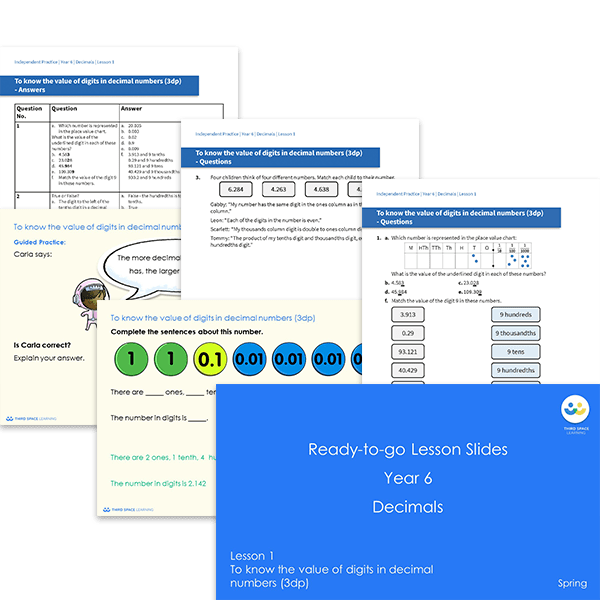# Ready-to-go lessons Year 6 Decimals (Spring Block 1) Slides and Worksheets

Decimals ready-to-go lessons for Year 6 includes 9 PowerPoints with matching worksheets ready for you to use with a class. These resources follow a mastery approach to mathematics teaching, similar to schemes such as White Rose Maths and Mathematics Mastery. The PowerPoints are fully editable with detailed notes for every teaching slide. Each associated worksheet is divided into three parts with a range of activities to complete across the block.

This Year 6 Decimals pack contains 9 fully editable PowerPoints and 9 worksheets.

The slides and worksheets cover:

• Knowing the value of digits in decimal numbers
• Multiplying and dividing by 10, 100 and 1,000
• Multiplying and dividing a decimal by an integer
• Dividing to solve problems
• Writing decimals as fractions
• Converting fractions to decimals

Year 6

Decimals